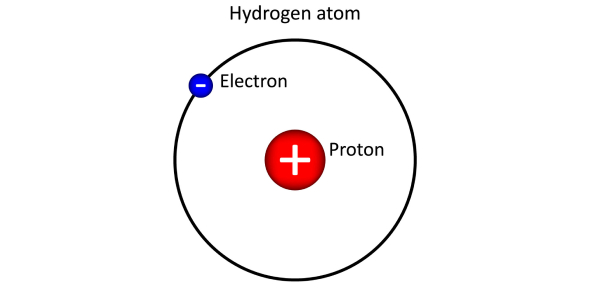# Models For Hydrogen Atom

9 Questions | Total Attempts: 269SettingsObjectives · Connect the importance of inference from experimental data. · Explain the concept of energy absorption and energy emission. · Identify the significance of only specific wavelengths of light being absorbed or emitted. Open the “Models of the Hydrogen Atom” simulation to the left Turn the light on, if you leave the light controls to white it will produce photons at all wavelength, if turn it to monochromatic then it will only produce photons at one wavelength. The spectrometer will measure photons deflected by the atom. Start with "experiment" results then when asked turn to "prediction"

• 1.
When determining how an atom works, scientists witnessed something similar to what you are witnessing now.  They then deduced how the atom must be organized.  What do you think is making the photons deflect?  Is every color deflected?
• A.

Yes

• B.

No

• C.

Maybe

• 2.
Make sure the spectrometer is on, let the spectrometer run for a couple minutes.  How many band are formed
• A.

No bands

• B.

1

• C.

3

• D.

5

• E.

7

• F.

9

• 3.
From this data does it appear that atom only interact with specific wavelengths?
• A.

Yes

• B.

No

• C.

Maybe

• 4.
Take a snap shot of the spectrometer (the camera button) then switch to predictions.  The billiard ball model is the Greek model. For some reason the spectrometer doesn't work for this prediction, but what kind of particles are been deflected by the atom?
• A.

Some

• B.

None

• C.

All

• D.

Just specfic photons

• 5.
Are the results from the billiard ball model the same for the "real" experiment?
• A.

Yes

• B.

No

• C.

Maybe

• 6.
Change to the plum pudding model and reset the spectrometer, let the simulation run while collecting data on the spectrometer.  Does the data from the plum pudding prediction match the data from the "real" experiment
• A.

Yes

• B.

No

• C.

Maybe

• 7.
The solar system model just blows up so lets not go there.  The next model is the Bohr model, reset the spectrometer and let the simulation run while collecting data.  Does the data from the Bohr model prediction match the data from the "real" experiment
• A.

Yes

• B.

No

• C.

Maybe

• 8.
The next model is the de Broglie model, reset the spectrometer and let the simulation run while collecting data. The de Broglie model is very close to the "real" experiment.  If you were unable to "see" into the infrared.  Would the data from the de Broglie prediction match the data from the "real" experiment
• A.

Yes

• B.

No

• C.

Maybe

• 9.
The last model predicts the band collected from the "real" experiment
• A.

True

• B.

False

Related TopicsBack to top